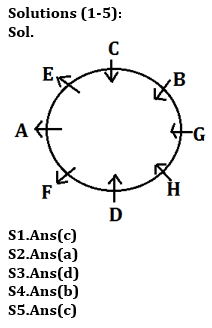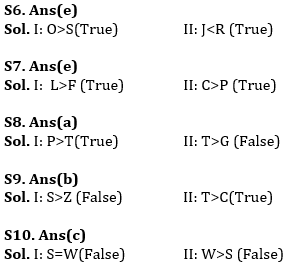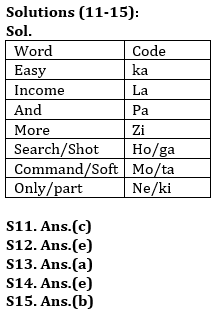Latest Banking jobs   »   Reasoning Ability Quiz For ESIC- UDC,...

# Reasoning Ability Quiz For ESIC- UDC, Steno, MTS Prelims 2022- 22nd January

Directions (1-5): Study the information carefully and answer the questions given below.
Eight persons A, B, C, D, E, F, G and H are sitting in a circular table. Some of them face towards the center and some of them face outside the center but not necessary in same order. G sits second to the right of D. Three persons sit between G and A. Immediate neighbor of E face opposite direction to each other’s. C sits second to the right of G. H sits second to the left of the one who is third to the left of G. F sits second to the left of H, who face inside. B does not sit opposite to H. F sits third to the right of C. C does not sit immediate left of E. The persons who face inside are more than the persons who face outside.

Q1. How many persons face inside?
(a) One
(b) Four
(c) More than Four
(d) Three
(e) None of these

Q2. Who among the following sits third to the right of H?
(a) C
(b) E
(c) B
(d) F
(e) None of these

Q3. Who among the following sits third to the right of D?
(a) E
(b) G
(c) H
(d) B
(e) None of these

Q4. Four of the following five are alike in certain way based from a group, find the one that does Shot belong to that group?
(a) B
(b) A
(c) G
(d) H
(e) D

Q5. Who among the following sits third to the left of A?
(a) G
(b) F
(c) H
(d) C
(e) None of these

Direction (6-10): In these questions, relationship between different elements is show in the statements. The statements are followed by conclusions. Study the conclusions based on the given statements and select the appropriate answer:
(a) If only conclusion I follows.
(b) If only conclusion II follows.
(c) If either conclusion I or II follows
(d) If neither conclusion I nor II follows.
(e) If both conclusions I and II follow.

Q6. Statements: S≤W<O≥R≥G>D≥J
Conclusion I: O>S      II: J<R

Q7. Statements: Q≤L>P=F<E<R=C
Conclusion I: L>F       II: C>P

Q8. Statements: E>T=R<C≤P≥G
Conclusion I: P>T     II: T>G

Q9. Statements: Z<X<T=E>S>L=C
Conclusion I: S>Z      II: T>C

Q10. Statements: S=M≤ Q=I≤W>D
Conclusion I: S=W  II: W>S

Directions (11-15): Study the information and answer the following questions:
In a certain code language
‘Easy search Shot income’ is written as ‘ka la ho ga’,
‘Command and Soft Easy’ is written as ‘mo ta pa ka’,
‘income more only part’ is written as ‘zi la ne ki’ and
‘Command more Soft Easy’ is written as ‘zi mo ka ta’.

Q11. What is the code for ‘and’ in the given code language?
(a) mo
(b) ta
(c) pa
(d) ka
(e) None of these

Q12. What is the code for ‘Soft’ in the given code language?
(a) Only ta
(b) Only mo
(c) Either pa or mo
(d) Only pa
(e) Either mo or ta

Q13. What may be the possible code for ‘part only more’ in the given code language?
(a) ne ki zi
(b) mo zi ne
(c) ki ne mo
(d) mo zi ki
(e) xi ka ta

Q14. What is the code for ‘more income’ in the given code language?
(a) la ne
(b) ga la
(c) zi ka
(d) zi ki
(e) la zi

Q15. What is the code for ‘Easy’ in the given code language?
(a) ta
(b) ka
(c) either ta or ka
(d) zi
(e) mo

Solutions